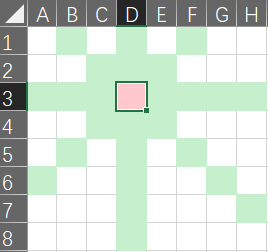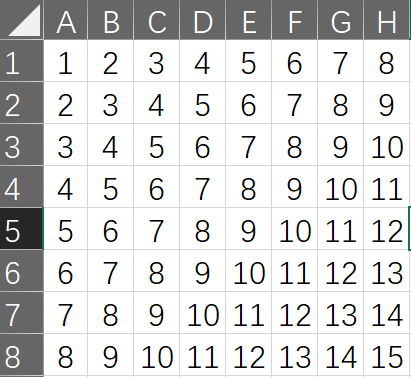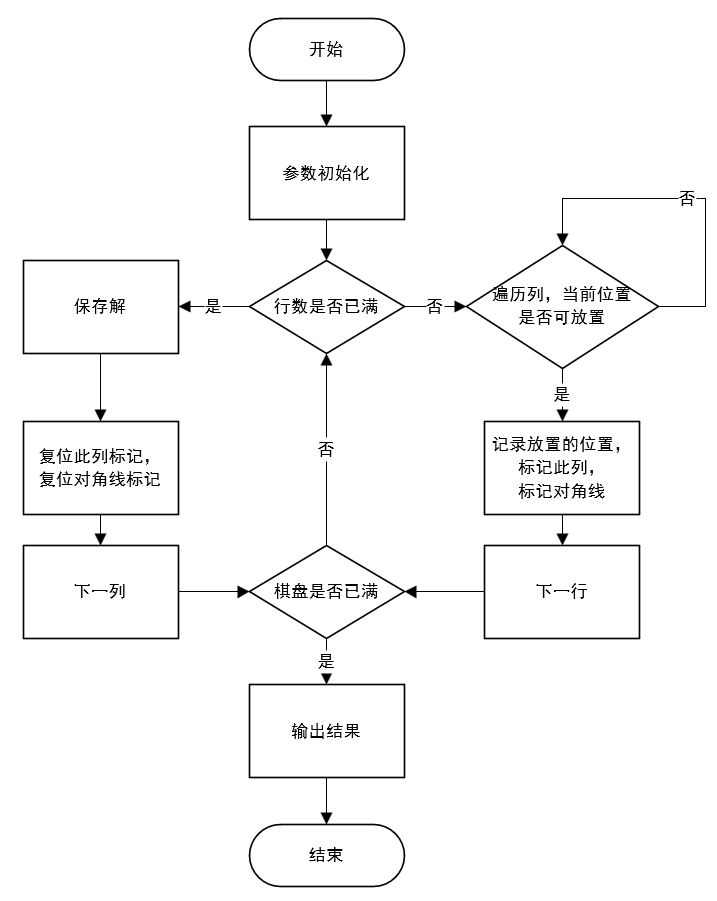• 八皇后问题的递归求解 C经典算法之一。值得学习。。。
• ## 八皇后问题-递归求解

千次阅读 多人点赞 2019-03-25 15:40:29
八皇后问题 国际象棋的棋盘上，按照国际象棋的规则，摆放8个皇后，使之“和平共处”。如图所示，3-D上有一个皇后，则绿色区域中都不能再放置皇后了。 最暴力的方法就是使用八个for，但是很明显，这种方法效率...
八皇后问题
在国际象棋的棋盘上，按照国际象棋的规则，摆放8个皇后，使之“和平共处”。如图所示，在3-D上有一个皇后，则绿色区域中都不能再放置皇后了。
最暴力的方法就是使用八个for，但是很明显，这种方法效率太低。对于放置了皇后的位置，仔细观察棋盘可以发现每一列（行）只能有一个皇后，每一个主（次）对角线上也只能有一个皇后，这样需要标记：行-row，列-col，主对角线-(n+row-col)，次对角线-(row+col)
注意：关于对角线的一点说明：
这里以次对角线为例：数字相同的表示一条次对角线，所以对于一个8x8的棋盘来说，一共有2x8-1条次对角线。流程图：基于流程图，利用递归的思想，c++实现如下，这里的皇后的个数是在主函数中设置的8，个数可以改，但意义不大。。。。。
在递归回溯的过程中清空了标记，是为了继续搜索，找到所有的解。
#include <iostream>
#include <vector>

using namespace std;

class EightQueen {
public:
EightQueen(int nQueen) {
this->nQueen = nQueen;
inColumn.resize(nQueen, false);
mainDiagonal.resize(2 * nQueen - 1, false);
minorDiagonal.resize(2 * nQueen - 1, false);
}
~EightQueen() {}
int process() {
int *path = new int[nQueen];
calculate(path, 0);
delete[] path;
return 0;
}
void calculate(int *path, int row) {
if (row == nQueen) {
solution.push_back(vector<int>(path, path + nQueen));
return;
}
for (int col = 0; col < nQueen; col++) {
if (canLay(row, col)) {//当前位置可放置
path[row] = col;//标记放置的位置
inColumn[col] = true;//当前皇后所在列
minorDiagonal[row+col] = true;//皇后所在位置的横纵坐标之和对应的次对角线
mainDiagonal[nQueen-1+row-col] = true;//皇后所在位置的横纵坐标之和对应的主对角线
calculate(path, row + 1);//下一行上的皇后
//break;//去掉搜索所有的解！
inColumn[col] = false;
minorDiagonal[row+col] = false;
mainDiagonal[nQueen-1+row-col] = false;
}
}
}
bool canLay(int row, int col) {
return !inColumn[col] && !minorDiagonal[row + col] && !mainDiagonal[nQueen - 1 + row - col];
}
void print() {
for (int i = 0; i < solution.size(); i++) {
cout << "solution " << i << " : " << endl;
for (int row = 0; row < nQueen; row++) {
for (int col = 0; col < solution[i][row]; col++) {
cout << "O ";
}
cout << "X ";
for (int col = solution[i][row]+1; col < nQueen; col++) {
cout << "O ";
}
cout << endl;
}
cout << endl << endl;
}
}
private:
int nQueen;
vector<bool> inColumn;
vector<bool> mainDiagonal;
vector<bool> minorDiagonal;
vector<vector<int> > solution;
};

int main()
{
EightQueen queen(8);
queen.process();
queen.print();
return 0;
}




展开全文递归
• 八皇后问题求解 c#代码例子 递归方法的完整代码
• 用回溯求解法求解八皇后问题，经典问题matlab实现，欢迎大家下载
• 八皇后问题 回溯法求解 Java程序设计 ！！！！！！ ！！！！！！！！！！ ！！
• 这是递归版本，随后会给出其他版本function Queens% 8皇后问题的递归法求解sol = 1; % 解的个数queen = zeros(8); % 8*8的棋盘saferows = true(1,8); % 用来表示每一行是否是安全位置safeleftdiag = true(1,15); % ...
这是递归版本，随后会给出其他版本function Queens% 8皇后问题的递归法求解sol = 1; % 解的个数queen = zeros(8); % 8*8的棋盘saferows = true(1,8); % 用来表示每一行是否是安全位置safeleftdiag = true(1,15); % 用来表示左对角是否安全，在同一个左对角上的元素满足i1+j1 = i2 + j2;saferightdiag = true(1,15);% 用来表示左对角是否安全，在同一个右对角上的元素满足i1-j1 = i2 - j2;trycol(1); % 检查第一列是否可以放置皇后% 该函数用来检查第col列是否可以方位皇后function trycol(col)% 对行循环，一行一行的去放for row = 1 : 8% 检查第row行第col列的位置是否安全if safe(row,col)% 如果安全，该位置被占据,该行和对角不安全[saferows(row),safeleftdiag(row + col - 1),saferightdiag(row - col + 8)]=deal(false);queen(row,col) = 1;% 如果是第8列，说明解完成，输出if col == 8fprintf('第%d个解\n',sol);disp(queen);sol = sol + 1;else % 否则，去试探下一列trycol(col + 1);end% 判断完该行，就去判断下一行，应该清除该行的占据信息和安全信息[saferows(row),safeleftdiag(row + col - 1),saferightdiag(row - col + 8)]=deal(true);queen(row,col) = 0;endendend% 该函数用来检查第row行第col列的位置是否安全function y = safe(row,col)% 检查该列的行，左对角，右对角是否安全y = saferows(row) & safeleftdiag(row + col - 1) & saferightdiag(row - col + 8);endend
展开全文• 是一种非常全新的任意皇后求解算法，代码实现上很有技巧！
• 八皇后问题（回溯法） **题目：**8×8格的国际象棋上摆放八个皇后，使其不能互相攻击，即任意两个皇后都不能处于同一行、同一列或同一斜线上，问有多少种摆法？ 代码： public class Queue8 { private int max =...
八皇后问题（回溯法）
**题目：**在8×8格的国际象棋上摆放八个皇后，使其不能互相攻击，即任意两个皇后都不能处于同一行、同一列或同一斜线上，问有多少种摆法？
代码：
public class Queue8 {

private int max = 8; // 表示棋盘大小
private static int count = 0;
private int[] arr = new int[max];// i表示第i+1行,也是第i+1个皇后，val=a[i]表示第val+1列 ,所以第i+1个皇后的坐标是（i+1,val+1）

public static void main(String[] args) {
Queue8 queue8 = new Queue8();
queue8.check(0);
System.out.println("count=" + count);
}

// 判断满不满足八皇后的要求，不能同一行同一列，或者是对角线
private boolean judge(int n) {
for (int i = 0; i < n; i++) {
if (arr[i] == arr[n] || Math.abs(n - i) == Math.abs(arr[n] - arr[i])) { // 不同行同列或者同一条线上的置为false
return false;
}
}

return true;

}

private void print() {
count++;// 统计多少种解法
for (int i = 0; i < max; i++) {
System.out.print(arr[i] + "  ");
}
System.out.println();

}

private void check(int n) {
if (n == max) {// n=max 表示递归结束
print();
return;
}

for (int i = 0; i < max; i++) {
arr[n] = i;// 给存储矩阵赋值

if (judge(n)) {// 判断第n+1行满足不满足条件,不满足则回溯到上面的循环
check(n + 1);// 满足继续深入递归
}
}
}

}




展开全文递归法 算法
•算法
• 八皇后问题是一个古老而著名的问题，是回溯算法的典型例题。该问题是十九世纪著名的数学家高斯1850年提出：8×8格的国际象棋上摆放八个皇后，使其不能互相攻击，即任意两个皇后都不能处于同一行、同一列或同一斜线...
八皇后问题是一个古老而著名的问题，是回溯算法的典型例题。该问题是十九世纪著名的数学家高斯1850年提出：在8×8格的国际象棋上摆放八个皇后，使其不能互相攻击，即任意两个皇后都不能处于同一行、同一列或同一斜线上，问有多少种摆法。

高斯认为有76种方案，1854年在柏林的象棋杂志上不同的作者发表了40种不同的解，后来有人用图论的方法解出92种结果。

算法分析：数组a、b、c分别用来标记冲突：
(1) 数组a代表列冲突，从a~a代表第0列到第7列，如果某列上已经有皇后，则为1，否则为0；
(2) 数组b代表主对角线冲突，为b[i-j+7]，即从b~b，如果某条主对角线上已经有皇后，则为1，否则为0；
(3) 数组c代表从对角线冲突，为c[i+j]，即从c~c，如果某条从对角线上已经有皇后，则为1，否则为0。

// eightQueen.cpp : 定义控制台应用程序的入口点。
//
#include "stdafx.h"
#include <iostream>
using namespace std;
#include <stdio.h>
/*本程序版权归http://www.loujing.com/所有，转载请注明出处*/
static char Queen;
static int a;
static int b;
static int c;
static int iQueenNum=0;  //记录总的棋盘状态数
void qu(int i);          //参数i代表行
int main()
{int iLine,iColumn;/*棋盘初始化，空格为*，放置皇后的地方为@*/for(iLine=0;iLine<8;iLine++){a[iLine]=0;      //列标记初始化，表示无列冲突for(iColumn=0;iColumn<8;iColumn++)Queen[iLine][iColumn]='*';}/*主、从对角线标记初始化，表示没有冲突*/for(iLine=0;iLine<15;iLine++)b[iLine]=c[iLine]=0;qu(0);return 0;
}
void qu(int i)
{int iColumn;for(iColumn=0;iColumn<8;iColumn++){if(a[iColumn]==0&&b[i-iColumn+7]==0&&c[i+iColumn]==0)  //如果无冲突{Queen[i][iColumn]='@';  //放皇后a[iColumn]=1;           //标记，下一次该列上不能放皇后b[i-iColumn+7]=1;       //标记，下一次该主对角线上不能放皇后c[i+iColumn]=1;         //标记，下一次该从对角线上不能放皇后if(i<7) qu(i+1);        //如果行还没有遍历完，进入下一行else                    //否则输出{/*输出棋盘状态*/int iLine,iColumn;printf("第%d种状态为：\n",++iQueenNum);for(iLine=0;iLine<8;iLine++){for(iColumn=0;iColumn<8;iColumn++)printf("%c  ",Queen[iLine][iColumn]);printf("\n");}printf("\n\n");}/*如果前次的皇后放置导致后面的放置无论如何都不能满足要求，则回溯，重置*/Queen[i][iColumn]='*';a[iColumn]=0;b[i-iColumn+7]=0;c[i+iColumn]=0;}}
}

展开全文• 回溯法求解八皇后问题 文件名 定位 作用 main.py 主模块 程序入口，利用回溯法求解八皇后问题 draw.py 绘图模块 将8...
• 八皇后问题8X8格的国际象棋上摆放八个皇后，使其不能互相攻击，即任意两个皇后都不能处于同一行、同一列或同一斜线上，问有多少种摆法。 通过回溯算法求解，可以得到92种位置摆法，去掉等效位置实际上只有24种...
•代码
• #include #include #define N 8 using namespace std; int board[N+1],cnt;...bool judge(int l,int n)//判断第l行第n个位置放是否合法 { for(int i=1; i; ++i) if(board[i]==n||abs(board[i]-n)==abs(i-l))
• /*0808 Eight queens puzzle*/ #include #include void ShowBoard(int (*chess)); //Print a solution bool AttackCalculate(int (*chess),int row, int col);
• 题目描述 会下国际象棋的人都很清楚：皇后可以横、竖、斜线上不限步数地吃掉其他棋子。如何将 8 个皇后放在棋盘上（有8×8个方格），使它们谁也不能被吃掉！这就是著名的八皇后问题算法 递归
• 八皇后为题概述；解决八皇后为题的步骤；完整代码。
• 八皇后问题，是一个古老而著名的问题，是回溯算法的典型例题。这里提供一个C++语言的递归法的实现，代码已VS2008下编译通过。相关博文地址： http://blog.csdn.net/jocodeoe/article/details/7067955
• 通过VC++求解八皇后问题的小程序，希望对大家有所帮助！...